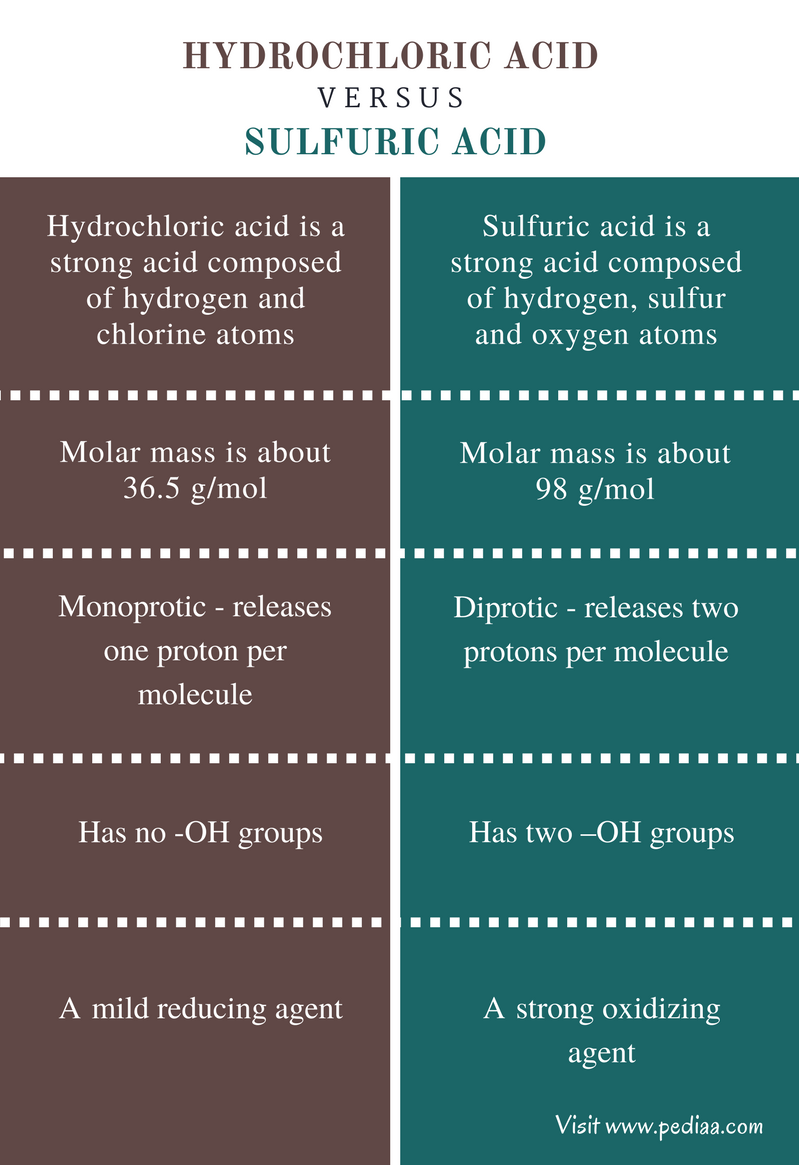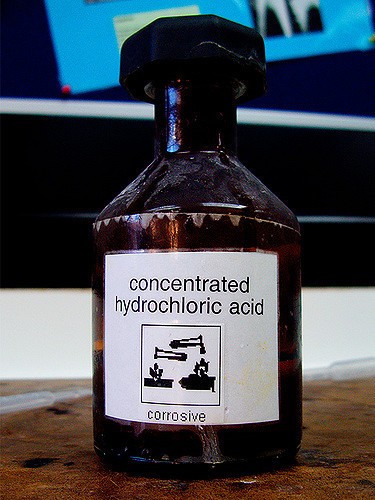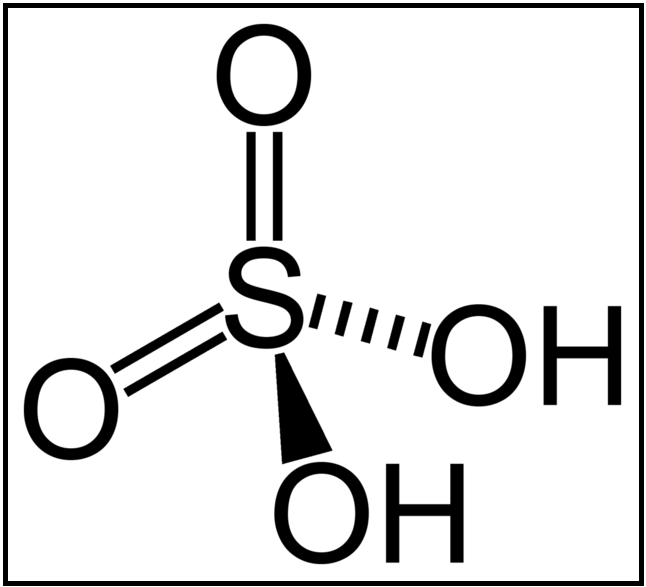Difference Between Hydrochloric Acid and Sulfuric Acid

Main Difference – Hydrochloric Acid vs Sulfuric Acid

Acids are molecules which are capable of donating protons or H+ ions to an aqueous solution. There are mainly two types of acids named strong acids and weak acids. Hydrochloric acid and sulfuric acid are two strong acids. The main difference between hydrochloric acid and sulfuric acid is that hydrochloric acid is monoprotic whereas sulfuric acid is diprotic.

Key Areas Covered

1. What is Hydrochloric Acid
– Definition, Properties
2. What is Sulfuric Acid
– Definition, Properties
3. What is the difference between Hydrochloric Acid and Sulfuric Acid
– Comparison of Key Differences

Key Terms: Chemical Formula, Diprotic, HCl, H2SO4, Hydrochloric Acid, Ionization, Monoprotic, Sulfuric AcidWhat is Hydrochloric Acid

A hydrochloric acid molecule is composed of hydrogen (H) and chlorine (Cl) atoms. The chemical formula of Hydrochloric acid is given as HCl. Molar mass of the HCl is about 36.5 g/mol. This acid is a colorless solution of HCl gas in water. Since it is a strong acid, HCl is corrosive and care should be taken when handling it.Figure 01: Hydrochloric Acid

HCl can donate one H+ ion per one HCl molecule, which means it is monoprotic (capable of releasing one proton). The reaction between HCl and water can be given as below.

HCl  +       H2O  →     Cl    +       H3O+

HCl has a large acid dissociation constant value (Ka). This acid is used in the determination of the concentration of base through titration. This is because it easily dissociates due to high Ka and Cl ion forms the salt with the cation of the base.

NaOH(aq)   +       HCl(aq)       →     NaCl(aq)     +       H2O(l)

In the above reaction, NaOH is the base and NaCl is the salt formed from the reaction.

Apart from that, hydrochloric acid is a mild reducing agent. It undergoes redox reactions with strong oxidizing agents such as MnO2.

MnO2(aq)     +       HCl(aq)      →    MnCl2(aq)   +       Cl2(g) +       H2O(l)

What is Sulfuric Acid

Sulfuric acid is a strong acid composed of hydrogen (H), oxygen (O) and sulfur (S) atoms. The molecular formula of sulfuric acid is given as H2SO4. The sulfuric acid is a diprotic acid. That means it can release two protons per molecule. The molar mass of sulfuric acid is about 98 g/mol. It is a very corrosive solution. But the corrosiveness depends on its concentration. Very low concentrations may not be harmful.Figure 01: Chemical Structure of Sulfuric Acid

The sulfuric molecule has two –O-H bonds which are very polar. Due to this polarity, the H atoms can easily be lost. The ionization of sulfuric acid occurs in two steps.

H2SO4(aq)    +       H2O(l)       →     HSO4(aq)   +       H3O+(aq)

HSO4(aq)    +       H2O(l)       →    SO4-2(aq)     +       H3O+(aq)

Sulfuric acid is a strong oxidizing agent in concentrated conditions. But when it is diluted, the oxidizing strength is reduced. For example, at higher temperatures, concentrated sulfuric acid can oxidize Br into Br2.

2HBr(aq)     +       H2SO4(aq)    →     Br2(aq)           +         SO2(aq)       +       2 H2O(l)

Difference Between Hydrochloric Acid and Sulfuric Acid

Definition

Hydrochloric Acid: Hydrochloric acid is a strong acid composed of hydrogen and chlorine atoms.

Sulfuric Acid: Sulfuric acid is a strong acid composed of hydrogen, sulfur and oxygen atoms.

Molar Mass

Hydrochloric Acid: The molar mass of hydrochloric acid is about 36.5 g/mol.

Sulfuric Acid: The molar mass of sulfuric acid is about 98 g/mol.

Proton Release

Hydrochloric Acid: Hydrochloric acid is monoprotic and releases one proton per molecule.

Sulfuric Acid: Sulfuric acid is diprotic and releases two protons per molecule.

Presence of –OH groups

Hydrochloric Acid: Hydrochloric acid has no -OH groups.

Sulfuric Acid: Sulfuric acid has two –OH groups.

Chemical Properties

Hydrochloric Acid: Hydrochloric acid is a mild reducing agent.

Sulfuric Acid: Sulfuric acid is a strong oxidizing agent.

Conclusion

The main difference between hydrochloric acid and sulfuric acid is that hydrochloric acid is monoprotic whereas sulfuric acid is diprotic. Both hydrochloric acid and sulfuric acid are very important in laboratory practices. Since they are acids, care should be taken when handling these solutions.

References:

1. “Hydrochloric acid.” Hydrochloric acid – New World Encyclopedia. N.p., n.d. Web. Available here. 27 June 2017.
2. “Chemical of the week – Sulfuric .” Chemical of the Week — Sulfuric Acid. N.p., n.d. Web. Available here. 27 June 2017.

Image Courtesy:

1. “Sulfuric-acid-2D” ( Public Domain) via Commons Wikimedia
2. “12 Mole Hydrochloric Acid” by maticulous (CC BY 2.0) via Flickr